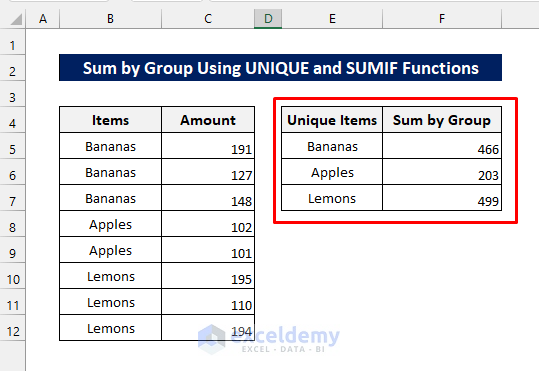# How to Sum by Group in Excel (4 Methods)Get FREE Advanced Excel Exercises with Solutions!

To sum by group means to add up values of a particular category. We often use it on numerous occasions in our daily lives. The summation of a group of values can be calculated in Excel in different ways. This article shows the 4 easiest ways to sum by group in Excel.

We will show you the 4 easiest ways to sum by group in this article. We will use the following dataset to illustrate these methods.## 1. Using IF and SUMIF Functions to Sum by Group

Suppose, you want to sum the amount for each item group in column D.Step 1: First enter the following formula in cell D5:

`=IF(B5=B4,"",SUMIF(B:B,B5,C:C))`

It gives the sum of the amounts for the first item category i.e. Bananas.Step 2: After that copy the formula to the cells below. It gives the sum of the amounts for each category as follows.## 2. Summing by Group Using Excel Subtotal Tool

You can also sum the amounts for each item category by using the Subtotal tool of Excel. To do that, apply the following steps.

Step 1: First, select the entire dataset as follows.Step 2: Then go to the Outline drop-down from the Data tab as shown below.Step 3: Now click on the Subtotal icon. This opens the Subtotal dialog box.Step 4: After that, check the ‘Amount’ field and hit OK.Step 5: Below, you will see the total for each category item obtained in the earlier method. Now, to get a more organized result, click on the ‘’ icons one by one. Clicking on the number ‘2’ icon also gives the same result.And now, you get the sum of the amounts for each group of items as follows.## 3. Using UNIQUE and SUMIF Functions to Sum by Group

Another alternative way to sum values by group is to use the UNIQUE and SUMIF functions. This method filters the unique items first. Then it gives the summation of the amounts for that unique item. To apply this method, follow the steps below.Step 1: Enter the following formula in cell E5:

`=UNIQUE(B5:B12,FALSE,FALSE)`

This filters the unique items in column E.Step 2: Now enter the following formula in cell F5.

`=SUMIF(B5:B12,E5,C5:C12)`

This gives the aggregate of the amounts for the first unique item.Step 3: Lastly, copy the formula down to the cells below to get the following result.## 4. Applying Excel PivotTable

Another easy but amazing way to get the summation of those amounts by item category is to use the PivotTable tool. For that, follow the steps below.

Step 1: At first, select the entire dataset as shown below. Then from the Insert tab, click on the PivotTable icon.Step 2: Now make sure the ‘Items’ and ‘Amount’ tables are checked and they are on the ‘Rows’ and ‘Values’ fields as follows. You can drag them to organize properly.Finally, you get the results as follows.## Things to Remember

• The dataset should be sorted before applying the first two methods. Using the Sort & Filter tool of Excel might be helpful to do so.
• You can use the SUMIF function only in the third method if you want the sum of one particular group of values at a time.
• Calculations were done on Office365. Look for the steps in your Microsoft Office version if you use a different one.
• PivotTable automatically detects the same group of data. So no need to worry about sorting your data while following the last method.

## Conclusion

Now, you know the easiest ways to sum values by category. We have used IF, SUMIF, and UNIQUE functions, the Subtotal and PivotTable tools of Excel in those methods. If you have further queries or suggestions, please share them with us in the comment section. We hope this article has helped you to understand how to sum values by group.

## What is ExcelDemy?

ExcelDemy Learn Excel & Excel Solutions Center provides free Excel tutorials, free support , online Excel training and Excel consultancy services for Excel professionals and businesses. Feel free to contact us with your Excel projects.

Tags:Md. Shamim Reza

Hello there! This is Md. Shamim Reza. Working as an Excel & VBA Content Developer at ExcelDemy. We try to find simple & easy solutions to the problems that Excel users face every day. Our goal is to gather knowledge, find innovative solutions through them and make those solutions available for everybody. Stay with us & keep learning.

We will be happy to hear your thoughtsAdvanced Excel Exercises with Solutions PDF